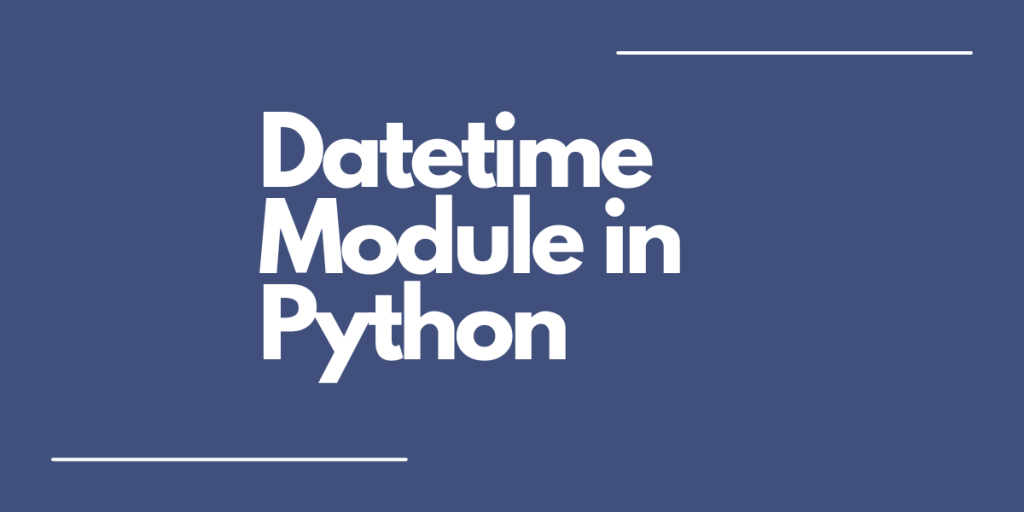# How to use the datetime module in Python?In Python, there is a module available called `datetime` that allows us to work with dates as well as time. It contains the date in the year, month, and day format and time in the hour, minute, and second format. This tutorial will show you how to work with this module.

In the `datetime` module, dates are represented in the below format:

``yyyy-mm-dd ``

And time is represented in the format:

``hh:mm:ss``

To compare dates and times, we use the usual comparison operators like `>=, <=, >, <, ==, !=`.
Consider two dates: d1 and d2.

Similarly, consider two times: t1 and t2.

All the above operations return a boolean value i.e. either ‘True’ or ‘False’, depending on whether the given condition is satisfied.
Before moving to date and time comparison, let’s first see some basic `datetime` methods.

## Examples of Python datetime methods

Let’s jump right into the examples of using the date time module here.

### 1. Get today’s date

```import datetime

print(datetime.date.today())
```

Output:

```2022-09-28
```

The date is represented in the yyyy-mm-dd format.

### 2. Get the current time

```from datetime import datetime

print(datetime.now().time())
```

Output:

```12:40:36.221835
```

The time is represented in the hh:mm:ss format.

## Date and time comparison

### 1. Check if one date is greater than the other one

```from datetime import datetime

#date in the format yyyy-mm-dd
date1 = datetime(2022, 5, 15)
date2 = datetime(2012, 4, 15)

print("Is date1 greater than to date2?: ", date1>date2)
```

Output:

```Is date1 greater than to date2?:  True
```

Here, the date1 is the 15th of May, 2022 and the date2 is the 15th of April, 2022. As date1 comes after date2 in the calendar, the output is true.

### 2. Check if one date is lesser than the other one

```from datetime import datetime

#date in the format yyyy-mm-dd
date1 = datetime(2022, 5, 15)
date2 = datetime(2022, 11, 16)

print("Is date1 less than to date2?: ", date1<date2)
```

Output:

```Is date1 less than to date2?:  True
```

In this example, date1 is again the 15th of May, 2022 and date2 is the 16th of November, 2022. As ‘May’ comes before ‘November’ in the same calendar year, the output is True.

### 3. Check if two dates are equal

```from datetime import datetime

#date in the format yyyy-mm-dd
date1 = datetime(2022, 5, 15)
date2 = datetime(2022, 4, 15)

print("Is date1 equal to date2?: ", date1==date2)
```

Output:

```Is date1 equal to date2?:  False
```

Here, the 15th of May, 2022 is not the same as the 15th of April, 2022. Hence, the output is False.

In the examples that we just saw, only the dates were given and not the time. Let’s learn how to compare only the dates or only the time if both date and time are given.

### 4. Comparing only the dates

```from datetime import datetime

#datetime in the format yyyy-mm-dd hh:mm:ss

#datetime1 -> date: 6 August, 2022 | time: 11:00:00 a.m.
datetime1 = datetime(2022, 8, 6, 11, 0, 0)

#datetime2 -> 21 March, 2022, | time: 2:45:31 p.m.
datetime2 = datetime(2022, 3, 21, 14, 45, 31)

#getting only the dates from datetime
date1 = datetime1.date()
date2 = datetime2.date()

print("Is date1 greater than date2?: ", date1>date2)
print("Is date1 less than date2?: ", date1<date2)
print("Is date1 equal to date2?: ", date1==date2)
```

Output:

```Is date1 greater than date2?:  True
Is date1 less than date2?:  False
Is date1 equal to date2?:  False
```

Here, we have used the `date()` method to extract only the date from the given datetime and then compared it using different comparison operators to get the output.

### 5. Comparing only the time

```from datetime import datetime

#datetime in the format yyyy-mm-dd hh:mm:ss

#datetime1 -> date: 6 August, 2022 | time: 11:00:00 a.m.
datetime1 = datetime(2022, 8, 6, 11, 0, 0)

#datetime2 -> 21 March, 2022, | time: 2:45:31 p.m.
datetime2 = datetime(2022, 3, 21, 14, 45, 31)

#getting only the time from datetime
time1 = datetime1.time()
time2 = datetime2.time()

print("Is time1 greater than time2?: ", time1>time2)
print("Is time1 less than time2?: ", time1<time2)
print("Is time1 equal to time2?: ", time1==time2)
```

Output:

```Is time1 greater than time2?:  False
Is time1 less than time2?:  True
Is time1 equal to time2?:  False
```

Similar to example 5, here we used the `time()` method from the datetime module to extract only the time from the given datetime.

## Summary

That’s all! We have learnt how to use the `datetime` module in Python for working with date and time as well.
If you want to learn about more different Python concepts, check out our other articles here!